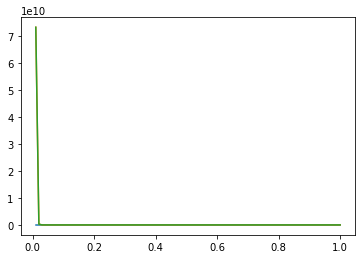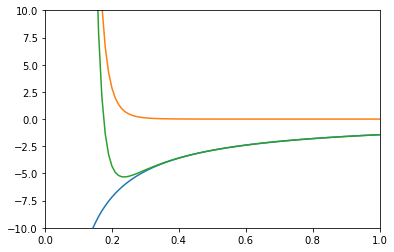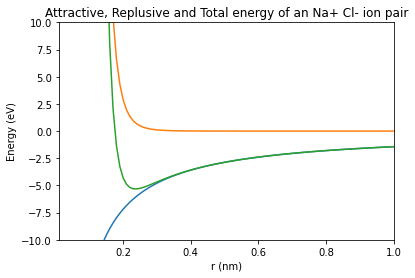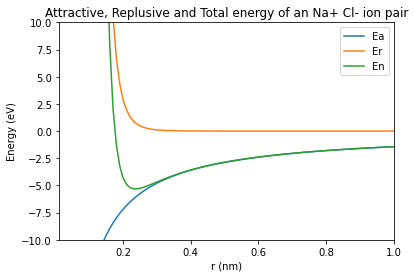Atoms in solid materials like steel and aluminum are held together with chemical bonds. Atoms of solid materials are more stable when they are chemically bonded together, and it takes energy to separate atoms which are joined together with a chemical bond. The bonding energy associated with a chemical bond describes the amount of energy needed to separate two atoms from their equilibrium positions to a substantial distance apart. In this post, we'll review how to build a line plot with Python and Matplotlib that describes the bond energy compared to the separation distance of a Na+ Cl- ion pair.

### Describe bond energy in terms of attractive and repulsive terms¶

For an Na+ Cl- pair, attractive and repulsive energies Ea and Er depend on the distance $r$ between the Na+and Cl- ions.

The attractive energy Ea and the repulsive energy energy Er of an Na+ Cl- pair depends on the inter-atomic distance, $r$ according to the following equations:

$$E_a = \frac{- 1.436}{r}$$$$E_r = \frac{7.32 \times 10^{-6}}{r^8}$$

The total bond energy, En is the sum of the attractive energy term Ea and the repulsive energy term Er:

$$E_n = E_a + E_r$$

On a single plot, we can graph Ea, Er and En vs. $r$ using Python and Matplotlib. Our plot will conform to the following parameters:

• The range of $r$ values should be between 0.01 and 1.00 in increments of 0.01
• The x-axis limits should be between $r$ = 0 and $r$ = 1.00
• The y-axis limits should be between E = -10 and E = 10

We will also include a plot title and axis labels with units. Each of the three lines on the plot will also be incorporated in a legend.

All of this can be accomplished with Python and Matplotlib. I use the Anaconda distribution of Python. See this post to see how to install Anaconda on Windows. If you use the Anaconda distribution of Python, Matplotlib is already installed. If you use Miniconda, a virtual environment or installed Python from Python.org, Matplotlib can be installed using the Anaconda Prompt or installed using a terminal and pip. The install command is below.

> conda install matplotlib numpy


or

$pip install matplotlib numpy  ### Import NumPy and Matplotlib¶ The first step to build our plot is to import NumPy and Matplotlib. We'll use NumPy to build an array that contains the distance values$r$and the energies Ea, Er and En. We'll use Matplotlib to build the plot. The necessary import commands are below. I am using a Jupyter notebook to build the plot. To see how open a Jupyter notebook, see this post. If you are using a Jupyter notebook, include the line %matplotlib inline. If you build your code in a .py-file, make sure to leave this line out as %matplotlib inline is not valid Python code. In : import numpy as np import matplotlib.pyplot as plt # if using a Jupyter notebook, include: %matplotlib inline  ### Create a NumPy array of r-values¶ Next, we will create a NumPy array of$r\$ values that starts at 0.01, ends at 1.00 and increments by 0.01. We'll create the array using NumPy's np.arange() function. The np.arange() function accepts three input arguments shown below.

np.arange(start,stop,step)


Note that Python counting starts at zero and ends at n-1. So if we want the last value in the array to be 1.00, we need to set our stop value as 1.01.

In :
r = np.arange(0.01,1.01,0.01)  # start, stop(exclusive), step


### Create arrays for the attractive energy term, the repulsive energy term and the total energy¶

Next we'll create arrays for the attractive energy term Ea, the repulsive energy term Er and the total energy En. We'll use the equations shown above and our array r to build these three arrays. Note that exponents are denoted in Python with the double asterisk symbol **, not the carrot symbol ^.

In :
Ea = -1.436/r              # attractive energy term
Er = (7.32e-6)/(r**(8))    # repulsive energy term
En = Ea + Er               # total energy


### Build a simple line plot¶

Now that we have the four arrays, we can build a simple line plot with Matplotlib. Each line on the plot is created using a pair of arrays. The first line uses the pair r, Ea, the second line on the plot uses the pair r, Er, and the third line on the plot is described by the pair r, En. Matplotlib's plt.plot() method accepts pairs of arrays (or pairs of lists) as shown below.

plt.plot(x1,y1,x2,y2,x3,y3....)


After the plt.plot() line, the command plt.show() shows the plot. These two lines have to be in the proper order.

In :
plt.plot(r,Ea,r,Er,r,En)
plt.show()We see a plot, but the plot looks like it only has one line on it. What's going on? We can only see one line because our y-axis has a very large range, between 0 and 1e10. If we limit the y-axis range, we'll be able to see all three line on the plot.

### Apply x-axis and y-axis limits¶

Next, we'll use Matplotlib's plt.xlim() and plt.ylim() commands to apply axis limits to our plot. A list or an array needs to be passed to these two commands. The format is below.

plt.xlim([lower_limit, upper_limit])

plt.ylim([lower_limit, upper_limit])


We'll limit our x-axis to start at 0.00 and end at 1.00. Our y-axis will be limited to between -10 and 10. The plt.plot() line needs to be before we customize the axis limits. The plt.show() line is included after we set the axis limits.

In :
plt.plot(r,Ea,r,Er,r,En)
plt.xlim([0.00, 1.00])
plt.ylim([-10, 10])
plt.show()Great! We see a plot with three lines. We can also see the y-axis starts at -10 and ends at 10. Next we'll add axis labels and a title to our plot.

### Axis labels and title¶

We can add axis labels to our plot with plt.xlabel() and plt.ylabel(). We need to pass a string enclosed in quotation marks ' ' as input arguments to these two methods. plt.title() adds a title to our plot.

In :
plt.plot(r,Ea,r,Er,r,En)
plt.xlim([0.01, 1.00])
plt.ylim([-10, 10])
plt.title('Attractive, Replusive and Total energy of an Na+ Cl- ion pair')
plt.xlabel('r (nm)')
plt.ylabel('Energy (eV)')
plt.show()We see a plot with three lines that includes axis labels and a title. The final detail we'll to add to our plot is a legend.

We can add a legend to our plot with the plt.legend() command. A list of strings needs to be passed to plt.legend() in the form below. Note the parenthesis, square brackets, and quotation marks.

plt.legend(['Entry 1', 'Entry 2', 'Entry 3'])


The plt.legend() line needs to be between the plt.plot() line and the plt.show() line just like our other plot customizations.

In :
plt.plot(r,Ea,r,Er,r,En)
plt.xlim([0.01, 1.00])
plt.ylim([-10, 10])
plt.title('Attractive, Replusive and Total energy of an Na+ Cl- ion pair')
plt.xlabel('r (nm)')
plt.ylabel('Energy (eV)')
plt.legend(['Ea','Er','En'])
plt.show()We see a plot with three lines, axis labels, a title, and a legend with three entries. There is one last step. In case we want to include the plot in a report or presentation, it would be nice to save the plot as an image file.

### Save the plot as an image file¶

If we want to include the plot in a Word document or a PowerPoint presentation, we can just right-click on the plot and select copy image or save image as. We can also save our plot programmatically with the plt.savefig() method. The plt.savefig() method takes a couple arguments including the image file name and the image resolution. Matplotlib infers the filetype based on the filename. The general format is below.

plt.savefig('filename.ext', dpi=resolution)


We'll save our plot as energy_vs_distance_curve.png with a resolution of 72 dpi (dots per inch). You could use a higher resolution in a printed document, such as200 dpi, but for a webpage 72 dpi is fine. The plt.savefig() line needs to be after the plt.plot() line and all the lines of customization, but before the plt.show() line.

The code below builds the plot and saves the plot as a separate image file.

In :
plt.plot(r,Ea,r,Er,r,En)
plt.xlim([ 0.01, 1.00])
plt.ylim([-10, 10])
plt.title('Attractive, Replusive and Total energy of an Na+ Cl- ion pair')
plt.xlabel('r (nm)')
plt.ylabel('Energy (eV)')
plt.legend(['Ea','Er','En'])
plt.savefig('energy_vs_distance_curve.png', dpi=72)
plt.show()### Summary¶

In this post, we created a plot with Python and Matplotlib. The plot contained three lines, axis labels, a title, and a legend. We also saved the plot to a separate image file. The Matplotlib commands we used to build the plot are summarized below.

Matplotlib method Description
plt.plot(x,y) construct a line plot
plt.xlabel('x label') x-axis label
plt.ylabel('y label') y-axis label
plt.title('title') plot title
plt.xlim([0, 10]) x-axis limits
plt.ylim([-5, 5]) y-axis limits
plt.legend(['line 1', 'line 2', 'line 3']) legend entries
plt.savefig('plot.png', dpi=72) save plot as an image file